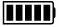ECE4253 Digital Communications Department of Electrical and Computer Engineering - University of New Brunswick, Fredericton, NB, Canada

# Galois Field GF(2) Calculator

Binary values representing polynomials in GF(2) can readily be manipulated using the rules of modulo 2 arithmetic on 1-bit coefficients. This online tool serves as a polynomial calculator in GF(2). Be aware that this is not an ordinary binary calculator.

 Model C-172 POLYNOMIAL CALCULATORA:

B:

 A + B A - B A × B A / B

Discussion  Polynomials  MATLAB

Enter binary values for two input operands and choose an operation. Select Polynomials to see the result in polynomial form. Select Discussion to see a detailed explanation of the underlying arithmetic.

``` 1100101
--------- = 1001, Remainder = 000
1101```

## Detailed Calculation

 ``` 1001 --------- 1101 ) 1100101 1101 ---- 001101 1101 ---- 000```

# MATLAB Example

Calculations in GF(2) are performed by MATLAB functions found within the Communications Toolbox.

>> a = gf( [1 1 0 0 1 0 1] );

>> b = gf( [1 1 0 1] );

>> [q,r] = deconv(a,b) % deconvolution is equivalent to division

q = GF(2) array.

Array elements =

1      0      0      1

r = GF(2) array.

Array elements =

0

 2023-06-06 16:13:47 ADT Last Updated: 2010-04-29 Richard Tervo [ tervo@unb.ca ]